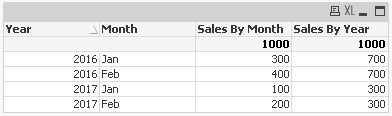# New to Qlik Sense

Discussion board where members can get started with Qlik Sense.

New Contributor III

## How to calculate different measures at different levels

Hi All,

I need to create a dashboard in the below format.

YearMonthSales by MonthSales by Year
2017Jan100500
2017Feb200500
2016Jan3001000
2016Feb4001000

In this dashboard the Sales by Month measure will be calculated based on Year and Month, i.e., in the example above, for Jan 2017 the sales is 100, for Jan 2016 sales is 300 etc. The Sales by Year value needs to calculated based on the year only, i.e., in the example above, the total sales for 2017 for all the months Jan-Dec is 500, the total sales for 2016 for all the months from Jan-Dec is 1000. For Sales by Year, the value always needs to show the sum for all 12 months irrespective of the months selected in the filter.

Can anyone please let me know how to calculate the Sales by Year value.

Thanks,

Rajarshi

4 RepliesMVP

## Re: How to calculate different measures at different levels

For Year 2017 Total sales for year is 300 and for Year 2016 is 700 but in sample it is

2017- 500

2016- 1000

New Contributor III

## Re: How to calculate different measures at different levels

Hi Anand,

In this part the user has selected only Jan and Feb in the filter. so data is shown only for the two months. But the Sales by Year value need to the sum of all months from Jan-Dec always irrespective of the months selected in the filter. So, I have put here the value for 2017 as 500 which is Jan+Feb+Mar+Apr+May+Jun+Jul+Aug+Sep+Oct+Nov+Dec.MVP

## Re: How to calculate different measures at different levels

Then make changes in the expression

Dim:- Year, Month

Expre:- Sum(Sale)

Expre:- =Sum( TOTAL <Year> Sales)

OutPut:-Find Attached

Highlighted
Honored Contributor III

## Re: How to calculate different measures at different levels

May be this Expression

If(Sum(Sales) <> 0,Sum({<Month>} TOTAL <Year> Sales))

Regards,

Antonio